magnetic field produced by the current in the secondary interacts with the magnetic field produced by the current in the primary. This interaction results from the mutual inductance between the primary and secondary windings. ">Custom SearchEFFECT OF A LOAD When a load device is connected across the secondary winding of a transformer, current flows through the secondary and the load. The magnetic field produced by the current in the secondary interacts with the magnetic field produced by the current in the primary. This interaction results from the mutual inductance between the primary and secondary windings. MUTUAL FLUX The total flux in the core of the transformer is common to both the primary and secondary windings. It is also the means by which energy is transferred from the primary winding to the secondary winding. Since this flux links both windings, it is called MUTUAL FLUX. The inductance which produces this flux is also common to both windings and is called mutual inductance. Figure 5-11 shows the flux produced by the currents in the primary and secondary windings of a transformer when source current is flowing in the primary winding. Figure 5-11. - Simple transformer indicating primary- and secondary-winding flux relationship.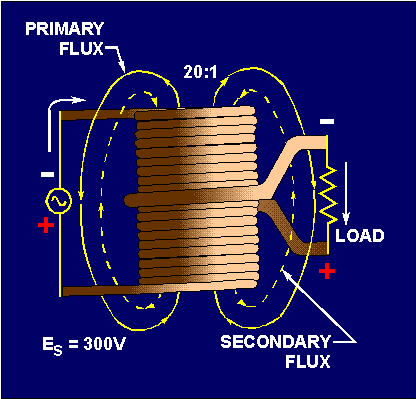When a load resistance is connected to the secondary winding, the voltage induced into the secondary winding causes current to flow in the secondary winding. This current produces a flux field about the secondary (shown as broken lines) which is in opposition to the flux field about the primary (Lenz's law). Thus, the flux about the secondary cancels some of the flux about the primary. With less flux surrounding the primary, the counter emf is reduced and more current is drawn from the source. The additional current in the primary generates more lines of flux, nearly reestablishing the original number of total flux lines. TURNS AND CURRENT RATIOS The number of flux lines developed in a core is proportional to the magnetizing force (IN AMPERE-TURNS) of the primary and secondary windings. The ampere-turn (I X N) is a measure of magnetomotive force; it is defined as the magnetomotive force developed by one ampere of current flowing in a coil of one turn. The flux which exists in the core of a transformer surrounds both the primary and secondary windings. Since the flux is the same for both windings, the ampere-turns in both the primary and secondary windings must be the same. Therefore: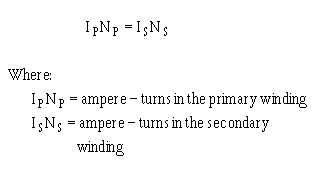By dividing both sides of the equation by IpN s, you obtain: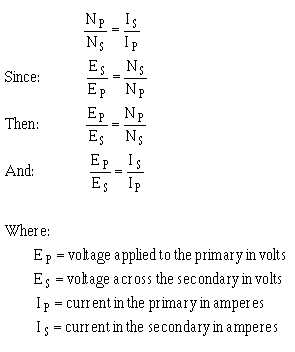Notice the equations show the current ratio to be the inverse of the turns ratio and the voltage ratio. This means, a transformer having less turns in the secondary than in the primary would step down the voltage, but would step up the current. Example: A transformer has a 6:1 voltage ratio. Find the current in the secondary if the current in the primary is 200 milliamperes.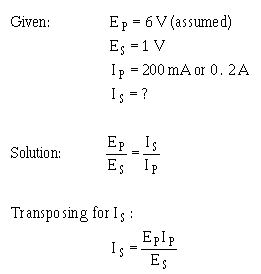The above example points out that although the voltage across the secondary is one-sixth the voltage across the primary, the current in the secondary is six times the current in the primary. The above equations can be looked at from another point of view. The expression is called the transformer TURNS RATIO and may be expressed as a single factor. Remember, the turns ratio indicates the amount by which the transformer increases or decreases the voltage applied to the primary. For example, if the secondary of a transformer has two times as many turns as the primary, the voltage induced into the secondary will be two times the voltage across the primary. If the secondary has one-half as many turns as the primary, the voltage across the secondary will be one-half the voltage across the primary. However, the turns ratio and the current ratio of a transformer have an inverse relationship. Thus, a 1:2 step-up transformer will have one-half the current in the secondary as in the primary. A 2:1 step-down transformer will have twice the current in the secondary as in the primary. Example: A transformer with a turns ratio of 1:12 has 3 amperes of current in the secondary. What is the value of current in the primary?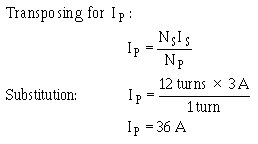Q.20 A transformer with a turns ratio of 1:3 has what current ratio? Q.21 A transformer has a turns ratio of 5:1 and a current of 5 amperes flowing in the secondary. What is the current flowing in the primary? (Assume no losses)Integrated Publishing, Inc. - A (SDVOSB) Service Disabled Veteran Owned Small Business